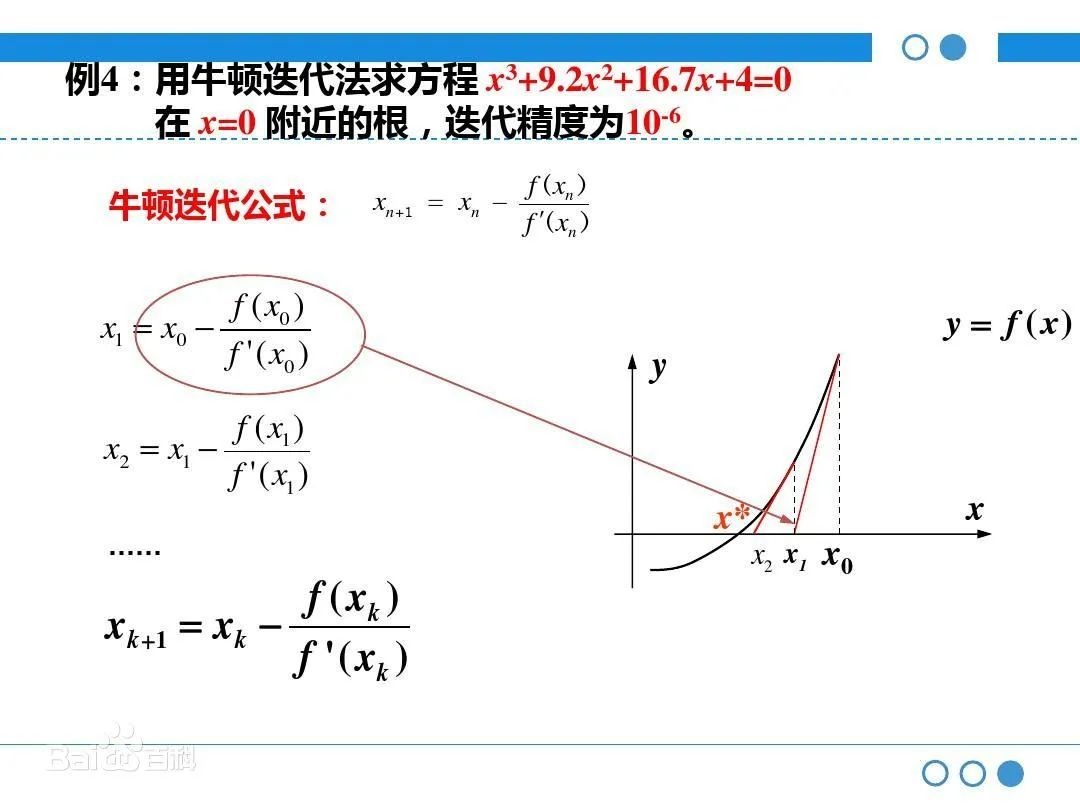• 转自利用牛顿迭代法自己写平方根函数sqrt 给定一个正数a，不用库函数求其平方根。 设其平方根为x，则有x2=a，即x2-a=0。设函数f(x)= x2-a,则可得图示红色的函数曲线。在曲线上任取一点(x0,f(x0))，...


转自利用牛顿迭代法自己写平方根函数sqrt

给定一个正数a，不用库函数求其平方根。

设其平方根为x，则有x2=a，即x2-a=0。设函数f(x)= x2-a,则可得图示红色的函数曲线。在曲线上任取一点(x0,f(x0))，其中x0≠0那么曲线上该点的切线方程为(1-1)

求该切线与x轴的交点得（1-2）

因为1-2式中x0作为分母，所以在之前限定了一下初始值不要选0。那么得到的这个与x轴的交点其实是最终要求得的x的一次逼近，我们再以这个x基准继续迭代就可以求得更逼近的x，至于逼近到什么时候才算完，这个取决于你自己设定的精度。整个过程的迭代只需要几步就可以求得最终的结果。

代码如下：

[cpp] view
plaincopy

double NewtonMethod(double fToBeSqrted)
{
double x = 1.0;
while(abs(x*x-fToBeSqrted) > 1e-5)
{
x = (x+fToBeSqrted/x)/2;
}

return x;
}

当然，从图中可以看出，当你所取的初始值的横坐标在红色曲线与x轴交点右边，即比最终的结果大时，比如选初始值x=a，我们可以将while语句里面的abs(x*x-fToBeSqrted)直接换成fToBeSqrted -x*x，这样可以省去abs的运算。当然这不能确保效率的提升，因为初始值的选取直接影响了迭代的次数。

转载于:https://www.cnblogs.com/noble/p/4144008.html
展开全文• 给定一个正数a，不用库函数求其平方根。...设函数f(x)= x2-a,则可得图示红色的函数曲线。在曲线上任取一点(x0,f(x0))，其中x0≠0那么曲线上该点的切线方程为  (1-1)  求该切线与x轴的交点得

给定一个正数a，不用库函数求其平方根。

设其平方根为x，则有x2=a，即x2-a=0。设函数f(x)= x2-a,则可得图示红色的函数曲线。在曲线上任取一点(x0,f(x0))，其中x0≠0那么曲线上该点的切线方程为(1-1)

求该切线与x轴的交点得（1-2）

因为1-2式中x0作为分母，所以在之前限定了一下初始值不要选0。那么得到的这个与x轴的交点其实是最终要求得的x的一次逼近，我们再以这个x基准继续迭代就可以求得更逼近的x，至于逼近到什么时候才算完，这个取决于你自己设定的精度。整个过程的迭代只需要几步就可以求得最终的结果。

代码如下：

[cpp] view
plaincopy

double NewtonMethod(double fToBeSqrted)
{
double x = 1.0;
while(abs(x*x-fToBeSqrted) > 1e-5)
{
x = (x+fToBeSqrted/x)/2;
}

return x;
}

当然，从图中可以看出，当你所取的初始值的横坐标在红色曲线与x轴交点右边，即比最终的结果大时，比如选初始值x=a，我们可以将while语句里面的abs(x*x-fToBeSqrted)直接换成fToBeSqrted -x*x，这样可以省去abs的运算。当然这不能确保效率的提升，因为初始值的选取直接影响了迭代的次数。

转载自：http://blog.csdn.net/wumuzi520/article/details/7026808

展开全文• 编写python函数实现求非负实数平方根，在shell里调用算法牛顿迭代法描述如下：函数代码调用函数 算法 牛顿迭代法 利用切线逼近曲线，求曲线零点，对于曲线 y=f(x)y = f(x)y=f(x),采用迭代的方法求其零点，在点（xn,f...
编写python函数实现求非负实数平方根，在shell里调用算法牛顿迭代法描述如下函数代码调用函数
算法
牛顿迭代法
利用切线逼近曲线，求曲线零点，对于曲线 $y = f(x)$,采用迭代的方法求其零点，在点$（x_n, f(x_n)）$处曲线切线方程：$y = f(x_n) + f'(x_n)(x - x_n)$,其与x轴交点横坐标即为下一个$x_n$，得到通用迭代公式：
$x_{n+1} = x_n + \frac{f(x_n)}{f'(x_n)}$
对于求已知参数 $x$ 的平方根，即求方程 $y^2 - x = 0$ 的解，代入即可解得：
求$x$平方根的迭代公式为：$y_{n+1}=\frac{y_n+\frac{x}{y_n}} {2}$
描述如下

对给定正整数$x$和允许误差$e$，令变量$y$取任意正整数值，如令 $y=x$；
如果 $y^2$与 $x$ 足够接近，即 $|y^2 - x| < e$，计算结束并把 $y$ 作为结果；
取 $z = \frac{y + \frac{x}{y}}{2}$ ;
将 $z$ 作为 $y$ 的新值，回到步骤1

函数代码
def sqrt(x):
y = 1.0
while abs(y * y - x) > 1e-6:
y = (y + x/y)/2
return y

调用函数
import sys
sys.path.append(r"D:\python")
import sqrt
y = sqrt.sqrt(5)
y



展开全文python 算法
• 要求X的平方根，那么必然存在一个实数N,使得N*N = X，所以可以转化为求函数f(x)=N²-X的零点问题。在曲线f(x)上，设N为f(x)=0的正根，那么设一个临近正根N的数r(为了方便就直接设r等于X)在r值对应的曲...


难度：medium题目描述：实现函数 int sqrt(int x)。计算并返回x的平方根示例：输入2，输出1.思路：这道题和C语言教材的那道题一样的。用牛顿迭代法。要求X的平方根，那么必然存在一个实数N,使得N*N = X，所以可以转化为求函数f(x)=N²-X的零点问题。 在曲线f(x)上，设N为f(x)=0的正根，那么设一个临近正根N的数r(为了方便就直接设r等于X)在r值对应的曲线上的点作切线，交X轴于新的一点r_new，此时r_new逐渐逼近正确零点值N，然后再在r_new值对应的曲线上的点作切线，又交X轴于新的一点r_new_new,此时r_new_new逐渐逼近正确零点值N...然后这样一直迭代下去，直到逼近正确值。如下图所示：(图来自网络，侵删)代码：import java.util.*;public class Solution {    public int sqrt (int x) {        //0和1特殊情况单独拿出来判断        if(x == 0 || x == 1){            return x;        }                //先设一个接近正确根的数r，为方便直接设等于x/2，减少迭代次数        long r = x/2;                //当r²>x的时候说明还没有找到        while(r * r > x){            //那么利用牛顿迭代公式求出新的x值进行迭代            //推导见最后            r = (r + x / r) / 2;        }                //当r²<=x的时候说明找到了直接返回        //根据示例直接返回的是整型        return (int) r;    }}//r = (r + x / r) / 2 公式来源://f(r)=r*r-x   (其中r为变量，x为常量)//根据牛顿迭代共公式：//r_new = r - f(r)/f'(r)//f'(r) = 2r//r_new = r - (r*r-x)/2r//r_new = r - r/2 + x/2r//r_new = r/2 + x/2r = (r + x / r) / 2

展开全文• 牛顿法：在实数域和复数域上近似求解方程的方法。 多数方程不存在求根公式，...设r是f(x) = 0的根，选取x0作为r初始近似值，过点（x0,f(x0)）做曲线y = f(x)的切线L，L的方程为y = f(x0)+f'(x0)(x-x0)，求出L与x...
• 百度了下“牛顿迭代法”，尼玛压根看不懂，内容中涉及到的曲线方程求导函数，以及求切线方程的知识都还给高中老师了，不看懂我心里难受，于是硬着头皮恶补相关知识。很多朋友把相关的知识写得很详细，关键在于理解...算法 迭代
• 关于使用牛顿迭代法求解LeetCode中计算X...这里给出牛顿法的思想：在迭代过程中，以直线代替曲线，用一阶泰勒展开式（即在当前点的切线代替原曲线），求直线与X轴的交点，重复这个过程直到收敛。话不多说，上图 现在分java leetcode 算法
• 参考链接：http://www.matrix67.com/blog/archives/361 https://www.zhihu.com/question/20690553 ... 核心思想：以直代曲。切线是曲线的线形逼近。 x^2 = a ---- 》 f(x) = x^2-a, 该函数导数f'(x)=2x. ...
• 要求sqrt(a），相当于求函数f(x)=x2-a的正根，牛顿法的思路是先随便初始化一个点(xn,f(xn))，然后求函数在该点的切线与x轴的交点xn+1，则xn+1是比xn更加接近所求解的，依次迭代下去就可以得到近似解。下面用一张图...
• 高数：对坐标的曲线积分这题怎么写?再问：可是答案是4a^2啊再问：奇怪再答：我感觉应该是你的答案错...dscosa=曲线对x求导除曲线对x求导的平方加曲线对y求导的平方之和的平方根高数：对坐标的曲线积分这是第二类曲线...
• 一、二分查找的前提目标函数的单调性（单调递增或者递减）存在上下界（bounded）能够通过索引访问（index accessible）二、代码模板left三、扩展69. x 的平方根​leetcode-cn.com# 二分查找方式解决 牛顿迭代法：在...
• 支持所有y=f[x,…]型及ρ=f[θ,…]型的(一元及多元)初等函数(包括N次函数、所有的(反)(双曲)三角函数、幂函数、指数函数、对数函数、绝对值、平方根、取整等,还可以自定义函数),所有的圆锥曲线(包括准线、渐近线)...
• 首先要明白，牛顿迭代法求的是函数和X轴的交点的横坐标，也就是我们说的根 1）那么第一步就是构建曲线了。 假设有一个数c，我们求它的平方根x，那么有一个等式，x^2 = c；挪到一边就是求 f = x^2 - c的根x 2）...sqrt java
• 因为吹水的能力不佳，所以要先打个草稿，今天的吹水过程大概是： ...牛顿迭代法就是在不断迭代的过程中逼近曲线的根，可以看做，它就是用来求曲线的根的。 所以它是怎么个迭代的过程，先上个动图： 牛顿迭代...算法
• sqrt（计算平方根值） 56 6.18 57 tan（取正切函数值） 57 6.19 57 tanh（取双曲线正切函数值） 57 7.用户组篇 58 7.1 58 endgrent（关闭组文件） 58 7.2 58 endpwent（关闭密码文件） 58 7.3 58 endutent（关闭utmp...linux c API
• ## R语言入门运行样例

千次阅读 2017-08-03 16:14:29
R语言入门运行样例 李东风 2016年6月27日 ...数学函数——平方根、指数、对数数学函数——取整数学函数——三角函数数学函数——反三角函数分布函数和分位数函数 ...函数曲线示例条形图示例散点图示例R软件自带的
• 结合声波时差测井数据与深侧向电阻率测井、自然伽马测井、密度测井数据之间的关系，数据拟合建立了声波时差模型，并采用压后压力降落G函数曲线、时间平方根曲线和双对数函数曲线预测了煤层压裂裂缝闭合压力；...
• 我们可以用几种不同的方法...我们可以通过假设它的二次、三次或平方根函数（或任何其他形式）来改变我们的假设函数的行为或曲线。 例如，我们的回归函数是然后，我们可以创建基于增加的变量X1，得到二次函数。或...
• 为了分析评估煤层气井压裂增产后的生产效果,以沁水盆地煤层气井为实例,通过G函数曲线、时间平方根曲线和双对数函数曲线预测煤层压裂裂缝闭合压力,并建立了煤层气井的地应力模型。基于该地应力模型的结果,将注入摩阻...
• 其实就是逼近的思想，例如我们要求a的平方根，首先令f(x)=x^2-a，那么我们的目的就是求得x使得f(x)=0，也就是求x^2-a这条曲线与x轴的交点，画图举例： 由函数f(x)=x^2-a，我们求导可以知道，函数上任意一点(x,y)...
• 实例1：三角函数曲线（1） 实例2：三角函数曲线（2） 实例3：图形的叠加 实例4：双y轴图形的绘制 实例5：单个轴窗口显示多个图形 实例6：图形标注 实例7：条形图形 实例8：区域图形 实例9：饼图的绘制 实例...matlab
• 牛顿迭代法求根 ...给定一个正数a，不用库函数求其平方根。...设函数f(x)= x2-a,则可得图示红色的函数曲线。在曲线上任取一点(x0,f(x0))，其中x0≠0那么曲线上该点的切线方程为 (1-1) ...
• 实例1：三角函数曲线（1） 实例2：三角函数曲线（2） 实例3：图形的叠加 实例4：双y轴图形的绘制 实例5：单个轴窗口显示多个图形 实例6：图形标注 实例7：条形图形 实例8：区域图形 实例9：饼图的绘制 实例10：阶梯...
• 三角函数曲线 4 实例1：三角函数曲线（1） 4 实例2：三角函数曲线（2） 4 实例4：双y轴图形的绘制 5 实例5：单个轴窗口显示多个图形 5 实例7：条形图形 6 实例8：区域图形 7 实例9：饼图的绘制 8 实例10：阶梯图 8 ...
• Andrew Ng有一个地方好像是错了，那种形状的曲线不是二次函数的形状，反倒是平方根函数形状。 Andrew 在讲神经网络的时候，给大家大致讲了一下神经元的知识。由于是拿英文讲的，满篇子的单词都不认识。所以去油管...
• 给定一个正数a，不用库函数求其平方根。 ...设函数f(x)= x2-a,则可得图示红色的函数曲线。在曲线上任取一点(x0,f(x0))，其中x0≠0那么曲线上该点的切线方程为  (1-1)  求该切线与x轴的交点得
• 实例50：绘制不同函数曲线的用户界面 实例51：修改函数曲线图颜色的用户界面 实例52：可设置函数曲线图视角的用户界面 实例53：可设置函数曲线光源的用户界面 实例54：添加效果 实例55：查询日期 实例56：图形效果...